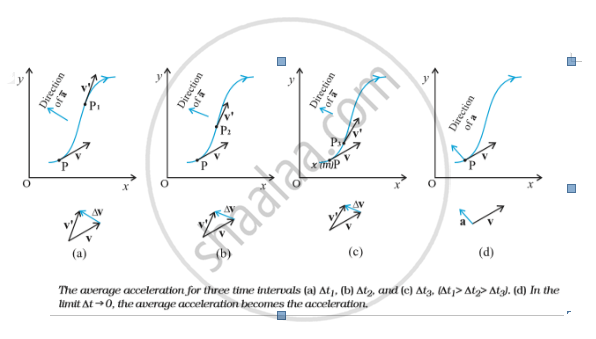# Motion in a Plane - Average Acceleration and Instantaneous Acceleration

## Notes

#### Acceleration:

The average acceleration a of an object for a time interval ∆t moving in x-y plane is the change in velocity divided by the time interval:
bar a = (Δv)/(Δt)=(Δv_x hat i + Δv_y hat j)/(Δt)=hat i (Δv_x)/(Δt) + hat j (Δv_y)/(Δt)
Or, bar a= bar a_x hat i + bar a_y bar j

The acceleration (instantaneous acceleration) is the limiting value of the average acceleration as the time interval approaches zero :
a = lim_(Δt→0) (Δv)/(Δt)
Since  Δv = Δv_x hat i + Δv_y hat j, we have
a= hat i lim_(Δt→0)(Δv_x)/(Δt) + hat j lim_(Δt→0)(Δv_y)/(Δt)
As in the case of velocity, we can understand graphically the limiting process used in defining acceleration on a graph showing the path of the object's motion. This is shown in figure below (a) to (d). P represents the position of the object at time t and P_1,P_2,P_3 positions after time Δt_1, Δt_2, Δt_3, respectively (Δt_1> Δt_2> Δt_3). The velocity vectors at points P, P_1,P_2,P_3 are also shown in figures (a), (b) and (c). In each case of Δt, Δv is obtained using traingle law of vector addition. By definition, the direction of average acceleration is the same as that of Δv. We see that as Δt decreases, the direction of Δv changes and consequently, the direction of the acceleration changes. Finally, in the limit Δt→0 [fig. (d)]. the average acceleration becomes the instantaneous acceleration and has the direction as shown.

Note that in one dimension, the velocity and the acceleration of an object are always along the same straight line (either in the same direction or in the opposite direction). However, for motion in two or three dimensions, velocity and acceleration vectors may have any angle between 0° and 180° between them.If you would like to contribute notes or other learning material, please submit them using the button below.

### Shaalaa.com

Motion in plane part 15 (Average acceleration) [00:04:37]
S
0%